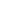My PegasusCART (0 ITEMS)eCourse: Module 1A - Basic Math[dd-c19-m01-t200]Description:
The math covered in this module is foundational to the physics covered in all of the later modules. With the exception of the CCI Science exam, which tests basic math concepts directly, the material taught in this module is tested indirectly on exams. Knowledge of basic mathematics facilitates answering physics test questions quickly and efficiently. In addition to the basics of how to deal with fractions, reciprocals, unit conversions, and equation manipulation, you will find significant emphasis placed on understanding the physical relationships between variables as expressed by mathematical equations. By learning the mathematical terminology and understanding the physical relationships between variables in physics equations, your ability to comprehend and answer test questions will significantly improve.retail: \$40.00
VIP: \$35.00